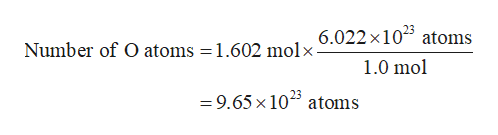# The molar mass of Al2(CO3)3 is 243.88 g/mol.How many atoms of oxygen are contained in 43.5 g of Al2(CO3)3?

Question
6 views

The molar mass of Al2(CO3)is 243.88 g/mol.How many atoms of oxygen are contained in 43.5 g of Al2(CO3)3?

check_circle

Step 1

The number of moles of Al2(CO3)3 present in the given sample is calculated using equation (1). In this equation, n represents the number of moles, m represents the mass, and M represents the molar mass of the species.

Step 2

Each Al2(CO3)3 molecule consists of 9 oxygen atoms. Therefore, the number of moles of oxygen atoms present in the given sample will be 9 times to the moles of Al2(CO3)3.

Step 3

One mole of a substance contains 6.022 × 1023 atoms which is known as Avogadro number. From...help_outlineImage Transcriptionclose6.022x105 atoms Number of O atoms = 1.602 molx- 1.0 mol 23 =9.65 x 10 atoms fullscreen

### Want to see the full answer?

See Solution

#### Want to see this answer and more?

Solutions are written by subject experts who are available 24/7. Questions are typically answered within 1 hour.*

See Solution
*Response times may vary by subject and question.
Tagged in

### Chemistry